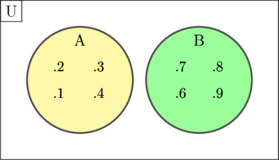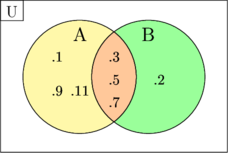# Venn Diagram

## Basic Concept

##### Disjoint Set and Joint Set

Disjoint set is two or more sets which have no elements in common

Example:

$$\text{U} = \lbrace 1, 2, 3, 4, 6, 7, 8, 9\rbrace$$

$$\text{A} = \lbrace 1, 2, 3, 4\rbrace$$

$$\text{B} = \lbrace 6, 7, 8, 9\rbrace$$

Set A and B are called disjoint setJoint set is two or more sets which have elements in common

Example:

$$\text{U} = \lbrace 1, 2, 3, 5, 7, 9, 11 \rbrace$$

$$\text{A} = \lbrace 1, 3, 5, 7, 9, 11\rbrace$$

$$\text{B} = \lbrace 2, 3, 5, 7\rbrace$$

Set A and B is joint set because they have elements in common {3, 5, 7}